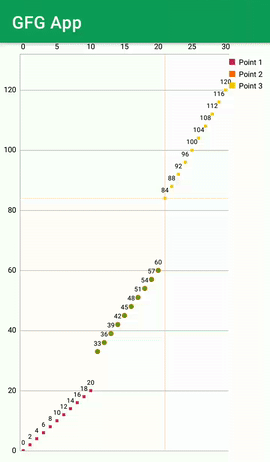# How to Create a Scatter Chart in Android to Represent Data?

• Last Updated : 17 Jan, 2021

If you are looking for showing a huge quantity of data and you are searching for a different UI design for the representation of this type of views. Then you can represent this view using a scatter chart. A Scatter chart is used to represent data. By using this scatter chart you can easily represent data in scattered form. In this article, we will see the implementation of the Scatter Chart in Android.

We will be building a simple chart in which we will be displaying a chart in which we will be displaying data. A sample GIF is given below to get an idea about what we are going to do in this article. Note that we are going to implement this project using the Java language.### Step by Step Implementation

Step 1: Create a New Project

To create a new project in Android Studio please refer to How to Create/Start a New Project in Android Studio. Note that select Java as the programming language.

implementation ‘com.github.PhilJay:MPAndroidChart:v3.1.0’

After adding this navigate to the build.gradle (Project) and add the below line to it inside the repositories section.

allprojects {

repositories {

// add below line in repositories section

maven { url ‘https://jitpack.io’ }

jcenter()

}

Step 3: Working with the activity_main.xml file

Go to the activity_main.xml file and refer to the following code. Below is the code for the activity_main.xml file.

## XML

 ```<``RelativeLayout``    ``xmlns:android``=``"http://schemas.android.com/apk/res/android"``    ``xmlns:tools``=``"http://schemas.android.com/tools"``    ``android:layout_width``=``"match_parent"``    ``android:layout_height``=``"match_parent"``    ``android:orientation``=``"vertical"``    ``tools:context``=``".MainActivity"``>`` ` `    ``<``com.github.mikephil.charting.charts.ScatterChart``        ``android:id``=``"@+id/chart1"``        ``android:layout_width``=``"match_parent"``        ``android:layout_height``=``"match_parent"` `/>`` ` ``

Step 4: Working with the MainActivity.java file

Go to the MainActivity.java file and refer to the following code. Below is the code for the MainActivity.java file. Comments are added inside the code to understand the code in more detail.

## Java

 `import` `android.os.Bundle;`` ` `import` `androidx.appcompat.app.AppCompatActivity;`` ` `import` `com.github.mikephil.charting.charts.ScatterChart;``import` `com.github.mikephil.charting.components.Legend;``import` `com.github.mikephil.charting.components.XAxis;``import` `com.github.mikephil.charting.components.YAxis;``import` `com.github.mikephil.charting.data.Entry;``import` `com.github.mikephil.charting.data.ScatterData;``import` `com.github.mikephil.charting.data.ScatterDataSet;``import` `com.github.mikephil.charting.interfaces.datasets.IScatterDataSet;``import` `com.github.mikephil.charting.utils.ColorTemplate;`` ` `import` `java.util.ArrayList;`` ` `public` `class` `MainActivity ``extends` `AppCompatActivity {``    ``// creating a variable for scatter chart``    ``private` `ScatterChart chart;`` ` `    ``@Override``    ``protected` `void` `onCreate(Bundle savedInstanceState) {``        ``super``.onCreate(savedInstanceState);``        ``setContentView(R.layout.activity_main);`` ` `        ``// initializing our scatter chart.``        ``chart = findViewById(R.id.chart1);``         ` `        ``// below line is use to disable the description ``        ``// of our scatter chart.``        ``chart.getDescription().setEnabled(``false``);``         ` `        ``// below line is use to draw grid background ``        ``// and we are setting it to false.``        ``chart.setDrawGridBackground(``false``);``         ` `        ``// below line is use to set touch ``        ``// enable for our chart.``        ``chart.setTouchEnabled(``true``);``         ` `        ``// below line is use to set maximum ``        ``// highlight distance for our chart.``        ``chart.setMaxHighlightDistance(50f);``         ` `        ``// below line is use to set``        ``// dragging for our chart.``        ``chart.setDragEnabled(``true``);``         ` `        ``// below line is use to set scale``        ``// to our chart.``        ``chart.setScaleEnabled(``true``);``         ` `        ``// below line is use to set maximum ``        ``// visible count to our chart.``        ``chart.setMaxVisibleValueCount(``200``);``         ` `        ``// below line is use to set ``        ``// pinch zoom to our chart.``        ``chart.setPinchZoom(``true``);``         ` `        ``// below line we are getting ``        ``// the legend of our chart.``        ``Legend l = chart.getLegend();``         ` `        ``// after getting our chart``        ``// we are setting our chart for vertical and horizontal``        ``// alignment to top, right and vertical.``        ``l.setVerticalAlignment(Legend.LegendVerticalAlignment.TOP);``        ``l.setHorizontalAlignment(Legend.LegendHorizontalAlignment.RIGHT);``        ``l.setOrientation(Legend.LegendOrientation.VERTICAL);``         ` `        ``// below line is use for ``        ``// setting draw inside to false.``        ``l.setDrawInside(``false``);``         ` `        ``// below line is use to set ``        ``// offset value for our legend.``        ``l.setXOffset(5f);``         ` `        ``// below line is use to get ``        ``// y-axis of our chart.``        ``YAxis yl = chart.getAxisLeft();``         ` `        ``// below line is use to set ``        ``// minimum axis to our y axis.``        ``yl.setAxisMinimum(0f);``         ` `        ``// below line is use to get axis``        ``// right of our chart``        ``chart.getAxisRight().setEnabled(``false``);``         ` `        ``// below line is use to get``        ``// x axis of our chart.``        ``XAxis xl = chart.getXAxis();``         ` `        ``// below line is use to enable``        ``// drawing of grid lines.``        ``xl.setDrawGridLines(``false``);``         ` `        ``// in below line we are creating an array list ``        ``// for each entry of our chart.``        ``// we will be representing three values in our charts.``        ``// below is the line where we are creating three``        ``// lines for our chart.``        ``ArrayList values1 = ``new` `ArrayList<>();``        ``ArrayList values2 = ``new` `ArrayList<>();``        ``ArrayList values3 = ``new` `ArrayList<>();``         ` `        ``// on below line we are adding data to our charts.``        ``for` `(``int` `i = ``0``; i < ``11``; i++) {``            ``values1.add(``new` `Entry(i, (i * ``2``)));``        ``}``         ` `        ``// on below line we are adding``        ``// data to our value 2``        ``for` `(``int` `i = ``11``; i < ``21``; i++) {``            ``values2.add(``new` `Entry(i, (i * ``3``)));``        ``}``         ` `        ``// on below line we are adding``        ``// data to our 3rd value.``        ``for` `(``int` `i = ``21``; i < ``31``; i++) {``            ``values3.add(``new` `Entry(i, (i * ``4``)));``        ``}``         ` `        ``// create a data set and give it a type``        ``ScatterDataSet set1 = ``new` `ScatterDataSet(values1, ``"Point 1"``);``         ` `        ``// below line is use to set shape for our point on our graph.``        ``set1.setScatterShape(ScatterChart.ScatterShape.SQUARE);``         ` `        ``// below line is for setting color to our shape.``        ``set1.setColor(ColorTemplate.COLORFUL_COLORS[``0``]);``         ` `        ``// below line is use to create a new point for our scattered chart.``        ``ScatterDataSet set2 = ``new` `ScatterDataSet(values2, ``"Point 2"``);``         ` `        ``// for this point we are setting our shape to circle``        ``set2.setScatterShape(ScatterChart.ScatterShape.CIRCLE);``         ` `        ``// below line is for setting color to our point in chart.``        ``set2.setScatterShapeHoleColor(ColorTemplate.COLORFUL_COLORS[``3``]);``         ` `        ``// below line is use to set hole ``        ``// radius to our point in chart.``        ``set2.setScatterShapeHoleRadius(3f);``         ` `        ``// below line is use to set color to our set.``        ``set2.setColor(ColorTemplate.COLORFUL_COLORS[``1``]);``         ` `        ``// in below line we are creating a third data set for our chart.``        ``ScatterDataSet set3 = ``new` `ScatterDataSet(values3, ``"Point 3"``);``         ` `        ``// inside this 3rd data set we are setting its color.``        ``set3.setColor(ColorTemplate.COLORFUL_COLORS[``2``]);``         ` `        ``// below line is use to set shape size``        ``// for our data set of the chart.``        ``set1.setScatterShapeSize(8f);``        ``set2.setScatterShapeSize(8f);``        ``set3.setScatterShapeSize(8f);``         ` `        ``// in below line we are creating a new array list for our data set.``        ``ArrayList dataSets = ``new` `ArrayList<>();``         ` `        ``// in below line we are adding all ``        ``// data sets to above array list.``        ``dataSets.add(set1); ``// add the data sets``        ``dataSets.add(set2);``        ``dataSets.add(set3);`` ` `        ``// create a data object with the data sets``        ``ScatterData data = ``new` `ScatterData(dataSets);``         ` `        ``// below line is use to set data to our chart``        ``chart.setData(data);``         ` `        ``// at last we are calling``        ``// invalidate method on our chart.``        ``chart.invalidate();``    ``}``}`

After adding the above code now run your app and see the output of the app.

### Output:

My Personal Notes arrow_drop_up